# RS Aggarwal Solutions for Class 9 Maths Chapter 11 - Circles Ex 11 C (11.3)

## RS Aggarwal Class 9 RS Aggarwal Solutions for Class 9 MathsChapter 11 - Circles Ex 11 C (11.3) Solutions Free PDF

Question 1:In the given figure ,PQ is a diameter of a circle with centre O . If ∠ PRQ = 65°,∠ SPR = 40° and ∠ PQM = 50°    ,     find ∠QPR ,∠ QPM and ∠ PRS.

Sol.

Consider the triangle Δ PRQ.

PQ is the diameter.

The angle in a semicircle is a right angle.

⇒ ∠ PRQ = 90°

By the angle sum property in Δ PRQ, we have

∠QPR + ∠PRQ +∠PQR = 180°

⇒ ∠QPR + 90° + 65° = 180°

⇒ ∠QPR = 180° – 155° = 25° …….(1)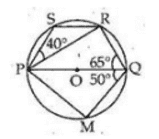Now Consider the triangle Δ PQM.

Since PQ is the diameter, ∠ PMQ = 90°’

Again by the angle sum property in Δ PRQ, we have

∠QPM+ ∠PMQ +∠PQM = 180°

⇒ ∠QPM + 90° + 50° = 180°

⇒ ∠QPR = 180° – 140° = 40°

∠QPS + ∠ SRQ = 180°

⇒ ∠QPR+ ∠RPS +∠PRQ + ∠ PRS = 180° [from (1)]

⇒ 25° + 40° + 90° + ∠ PRS = 180°

⇒       ∠ PRS = 180° – 155° = 25°

∴      ∠ PRS = 25°

Question 2: In the given figure ABCD is a cyclic quadrilateral whose diagonals intersect at P such that ∠ DBC = 60° and ∠ BAC = 40° , find

1. ∠ BCD

Sol.

(i) ∠ BDC = ∠ BAC = 40° [angles in the same segment]

In Δ BCD, we have

∠ BCD +∠ BDC + ∠ DBC =180°

∴ ∠ BCD +40° + 60° =180°

⇒ ∠ BCD = 180° -100° = 80°

∴ ∠ BCD = 80°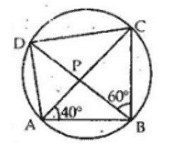(ii) Also ∠ CAD = ∠ CBD [angles in the same segment]

∠ CAD = 60° [∵ ∠ CBD = 60°]

Question 3: In the given figure, PQR is a diameter and PQRS is a cyclic quadrilateral. If ∠ PSR = 150°, find∠ RPQ

Sol.

∠ PSR + ∠ PQR = 180°

⇒ 150° + ∠ PQR = 180°

⇒ ∠ PQR = 180° – 150° = 30° …….(1)

Also, ∠ PQR = 90° …….(2) [angles in a semicircle]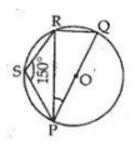Now in Δ PRQ, we have

∠ PQR + ∠ PRQ + ∠ RPQ = 180°

⇒ 30° + 90°+ ∠ RPQ = 180° [from (i) and (ii)]

⇒ ∠ RPQ = 180° – 120° = 60°

∴ ∠ RPQ = 60°

Question 4: In the given figure ABCD is a cyclic quadrilateral in which AB || DC . If       BAD = 100°  , find

1. ∠ BCD
3. ∠ ABC

Sol.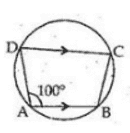(i) ∠ BCD + ∠ BAD = 180°

⇒ ∠ BCD + 100° = 180°

⇒ ∠ BCD = 180° – 100° = 80°

(ii) Also, ∠ ADC = ∠ BCD = 80°

∴ ∠ ACD = 80°

(iii) ∠ ABC = ∠ BAD = 100°

∠ ABC = 100°

Question 5: In the given figure O is the centre of the circle and arc ABC subtends an angle of 1300 at the centre . If  AB is extended to P , find ∠ PBC

Sol.

Take a point D on the major arc CA and join AD and DC

∴ ∠ 2 = 2∠ 1 [Angle subtended by an arc is twice the angle subtended by it on the circumference in the alternate segment.]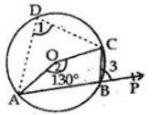∴ 130° = 2∠ 1

⇒ ∠ 1 = 65° …….(1)

∠ PBC = ∠1 [∵ Exterior angle of a cyclic quadrilateral interior opposite angle]

∴ ∠ PBC = 65°

Question 6: In the given figure ABCD is a cyclic quadrilateral in which AE is drawn parallel to CD and BA is produced . If∠ABC=92°  and ∠ EAF = 20°, find∠ BCD.

Sol.

∴ ∠ABC + ∠ ADC = 180°

⇒ 92° + ∠ ADC = 180°

⇒ ∠ ADC = 180° -92° = 88°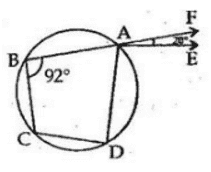Also, AE || CD

∴ ∠ BCD = ∠ DAF [∵ Exterior angle of a cyclic quadrilateral = interior opposite angle]

∴ ∠ BCD = ∠ EAD + ∠ EAF

= 88° + 20° [∵∠ FAE = 20° (given)]

= 108°

∴ ∠ BCD = 108°

Question 7: In the given figure , BD=DC and ∠ CBD = 30°, find m(∠ BAC)

Sol.

BD = DC

∴ ∠ BCD = ∠ CBD = 30°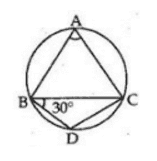In Δ BCD, we have

∠ BCD + ∠ CBD + ∠ CDB = 180°

⇒ 30° + 30° + ∠ CDB = 180°

⇒ ∠ CDB = 180° – 60°

= 120°

The opposite angles of a cyclic quadrilateral are supplementary.

ABCD is a cyclic quadrilateral and thus,

∠ CDB + ∠ BAC = 180°

= 180° -120° [∵∠ CDB = 120°]

= 60°

∴ ∠ BAC = 60°

Question 8: In the given figure , O is the centre of the given circle and measure of arc ABC is 1000. Determine ∠ ADC  and ∠ ABC .

Sol.

Angle subtended by an arc is twice the angle subtended by it on the circumference in the alternate segment.

Here arc ABC makes ∠ AOC = 100° at the center of the circle and ∠ ADC on the circumference of the circle.

∴ ∠ AOC = 2∠ ACD

⇒ ∠ ACD = ½ (∠AOC)

⇒ = ½ × 100° [∠ AOC = 100°]

⇒ ∠ ACD = 50°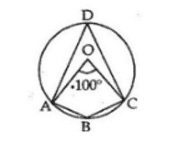The opposite angles of a cyclic quadrilateral are supplementary.

ABCD is a cyclic quadrilateral and thus,

∠ ACD + ∠ ABC = 180°

= 180° – 50° [∵ ∠ ADC = 50°]

= 130°

∴ ∠ ABC = 130°

∴ ∠ ACD = 50° and ∠ ABC = 130°

Question 9: In the given figure , Δ ABC is equilateral . Find

1. ∠ BDC
2. ∠ BEC

Sol.

Δ ABC is an equilateral triangle.

∴   Each of its angle is equal to 60°

⇒ ∠ BAC = ∠ ABC = ∠  ∠ ACB = 60°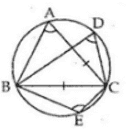(i) Angles in the same segment of a circle are equal.

∴ ∠ BDC = ∠ BAC

= 60° [∵ ∠ BAC = 60°]

(ii) The opposite angles of a cyclic quadrilateral are supplementary.

ABCE is a cyclic quadrilateral and thus,

∠ BAC + ∠ BEC = 180°

∠ BEC = 180° – 60° [∵ ∠ BAC = 60°]

= 120°

⇒ ∠ BEC = 120°

Question 10: In the adjoining figure, ABCD is a cyclic quadrilateral in which ∠ BCD = 100° and ∠ ABD = 50° , find ∠ ADB

Sol.

∠ A + ∠ C = 180° [The opposite angles of a cyclic quadrilateral are supplementary]

⇒ ∠ A + 100°= 180°

⇒ ∠ A = 180° – 100° = 80°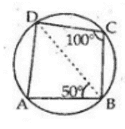Now in Δ ABD, we have

∠ A + ∠ ABD + ∠ ADB = 180°

⇒ 80° + 50° + ∠ ADB = 180°

⇒ ∠ ADB = 180° – 130° = 50°

Question 11: In the given figure , O is the centre of the circle and ∠ DAB = 150° find value of x and y

Sol.

O is the center of the circle and ∠ BOD = 150°

∴ Reflex   ∠ BOD = (360° – ∠ BOD)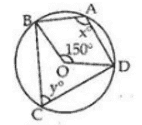= (360° – 150°) = 210°

Now, X = ½(reflex ∠ BOD)

= ½ × 210° = 105°

X = 105°

Again, X + Y = 180°

⇒ 105° + Y = 180°

⇒           Y = 180° – 105° = 75°

∴            Y = 75°

Question 12: In the given figure , O is the centre of the circle and ∠ DAB = 50° . Find value of x and y.

Sol.

O is the center of the circle and ∠ DAB= 50°

⇒ ∠ OBA = ∠ OAB = 50°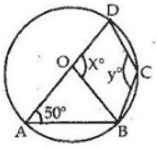In Δ OAB, we have

∠ OAB + ∠ OBA + ∠ AOB =180°

⇒   50° + 50° + ∠ AOB =180°

⇒    ∠ AOB =180° – 100° = 80°

Since, AOD is a straight line,

X = 180° – ∠ AOB

= 180° – 80° = 100°

∴ X = 100°

The opposite angles of a cyclic quadrilateral are supplementary.

ABCD is a cyclic quadrilateral and thus,

∠ DAB + ∠ BCD = 180°

∠ BCD = 180° – 50° [∵ ∠ DAB = 50°, given]

⇒ Y = 130°

Thus, X = 100° and Y = 130°.

Question 13: In the given figure sides AD and AB of cyclic quadrilateral ABCD are produced to E and F respectively. If ∠ CBF=130°  and ∠ CDE= X0 , find the value of x

Sol.

We know that in a cyclic quadrilateral exterior angle = interior angle.

∴ ∠ CBF = ∠ CDA = (180° – X)

⇒ 130° = 180° – X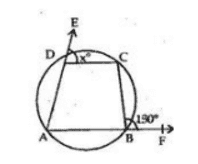⇒ X = 180° – 130° = 50°

X = 50°

#### Practise This Question

When can we say that we are not healthy?